# Design and Analysis of a Diamond-Shaped Antenna for Ultra-Wideband Applications

This example shows how to create the diamond-shaped ultra-wideband (UWB) antenna described in  with a modified ground plane. UWB antennas are in high demand for various applications, such as wireless communications, medical imaging, radar, and indoor positioning. This demand is due to their ability to enable a high data transmission rate and low power consumption.

The planar diamond-shaped monopole antenna structure described in  achieves an ultra-wide bandwidth by using its features, such as its small size, ease of fabrication, low power consumption, and easy integration with the RF system. These features make the antenna a suitable candidate for use in compact devices.

In this example, you design the proposed antenna on a 1.6 mm FR4 substrate with a dielectric constant of 4.4. This antenna consists of a diamond-shaped radiating patch, a modified feedline, and a semicircular ground plane. You then modify the conventional rectangular monopole antenna with beveled edges to form a diamond-shaped radiating patch.

#### Create Antenna Model

Define the length and width of the ground plane.

```gndL = 32e-3; gndW = 40e-3; % Define the board thickness. h = 1.6e-3; R_gnd = 15.6e-3; % Define the substrate. sub = dielectric("Name",{'FR4'},"EpsilonR",4.4,'Thickness',h); % Specify the widths of the traces. Wf = 3.1e-3; Wf1 = 1.2e-3; W3 = 13e-3; W2 = 29e-3; W1 = 22e-3; % Specify the lengths of the traces. Lf = 10e-3; Lf1 = 6e-3; L3 = 2e-3; L2 = 11e-3; L1 = 5e-3;```

Create the top-layer diamond-shaped antenna by passing the parameters and vertices to the `antenna.Polygon` object.

```xver = round([-Wf/2 -Wf/2 -Wf1/2 -W3/2 -W2/2 -W1/2 W1/2 W2/2 W3/2 Wf1/2 Wf/2 Wf/2],12); yver = round( [-gndW/2 -gndW/2+Lf -gndW/2+Lf+Lf1 -gndW/2+Lf+Lf1+L3 -gndW/2+Lf+Lf1+L3+L2 ... -gndW/2+Lf+Lf1+L3+L2+L1 -gndW/2+Lf+Lf1+L3+L2+L1 -gndW/2+Lf+Lf1+L3+L2 ... -gndW/2+Lf+Lf1+L3 -gndW/2+Lf+Lf1 -gndW/2+Lf -gndW/2],12); zver = zeros(1,size(yver,2)); ver = [xver',yver',zver']; UWB_ant = antenna.Polygon('Vertices',ver);```

Create the semicircular ground layer.

```cir = antenna.Circle('Radius',R_gnd,'Center',[0,-gndW/2]); rec = antenna.Rectangle('Length',gndL+2e-3,'Width',R_gnd,'Center',[0,-gndW/2-R_gnd/2]); rec1 = antenna.Rectangle('Length',0.5*R_gnd,'Width',0.5*R_gnd,'Center',[-gndL/2-(0.5*R_gnd)/2,-gndW/2+(0.5*R_gnd)/2]); rec2 = antenna.Rectangle('Length',0.5*R_gnd,'Width',0.5*R_gnd,'Center',[gndL/2+(0.5*R_gnd)/2,-gndW/2+(0.5*R_gnd)/2]); gnd = cir - (rec+rec1+rec2);```

Generate the UWB antenna model by using the `pcbStack` object with the assigned ground layer.

```ant = pcbStack; ant.BoardThickness = h; ant.BoardShape = antenna.Rectangle('Length',gndL,'Width',gndW); ant.Layers = {UWB_ant,sub,gnd}; ant.FeedLocations = [0,-gndW/2,1,3]; ant.FeedDiameter = Wf/2;```

Show the antenna.

```figure; show(ant);```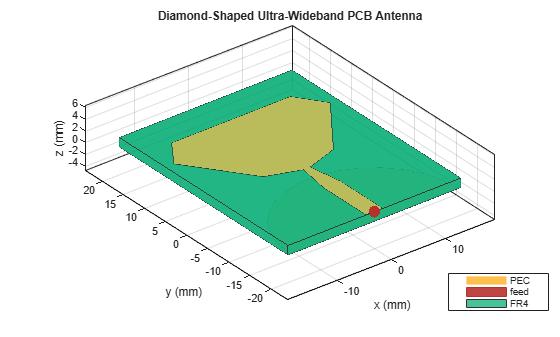#### Analyze Return Loss and Gain of Antenna

Mesh the structure using manual mesh mode with a maximum edge length of 0.003 m.

```figure; mesh(ant,'MaxEdgeLength',0.003);```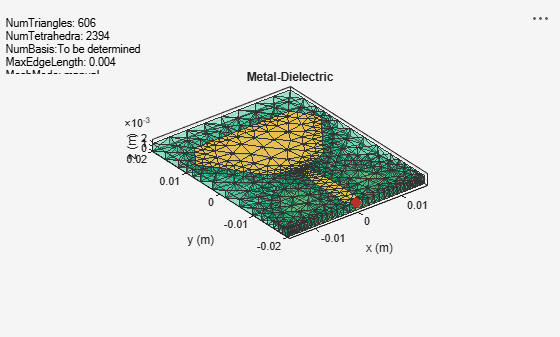Use the `sparameters` function to analyze the return loss of the structure in the frequency range of 0.1 GHz to 15 GHz.

```spar = sparameters(ant,linspace(0.1e9,15e9,51)); figure; rfplot(spar);```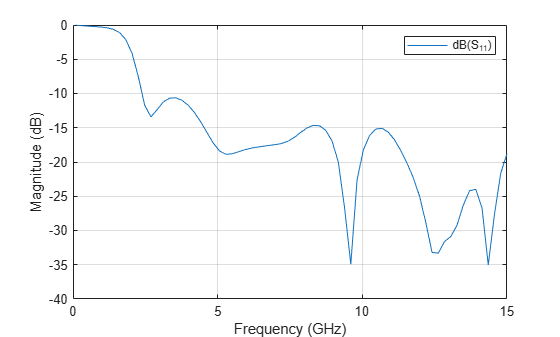The UWB diamond-shaped antenna exhibits a passband behavior in the frequency range of 2.5 GHz to 15 GHz with a return loss greater than -10 dB.

Analyze the radiation pattern of the wideband antenna at 4 GHz, 8 GHz, and 15 GHz within the frequency spectrum.

```figure; pattern(ant,4e9);```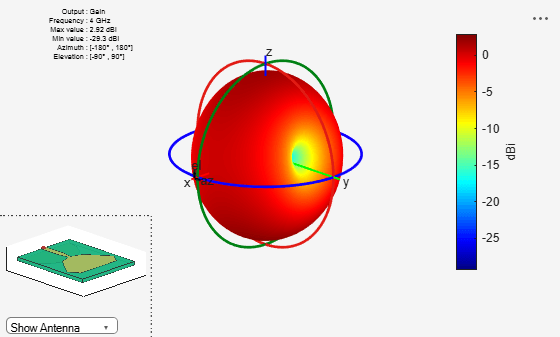```figure; pattern(ant,8e9);```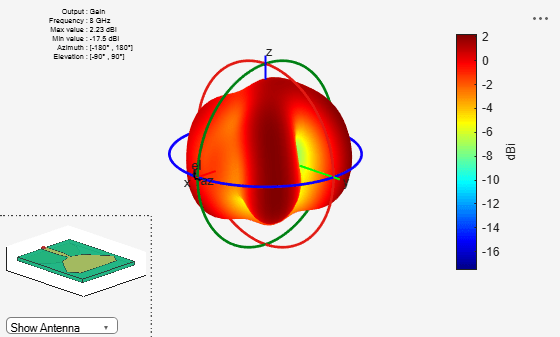```figure; pattern(ant,15e9);```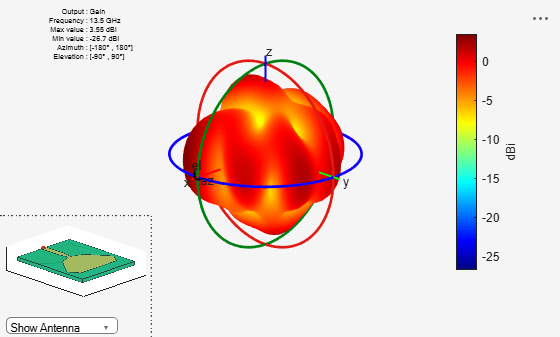The proposed antenna exhibits stable radiation characteristics with a gain of 3.3 dBi, 3.26 dBi, and 3.99 dBi at 4, 8, and 15 GHz, respectively.

### Conclusion

The diamond-shaped antenna achieves the enhanced ultra-wide bandwidth, in which the UWB frequency spectrum covers the range from 3.1 GHz to 10.6 GHz, with stable radiation characteristics. Because the proposed antenna has good UWB characteristics and a compact size, it is suitable for wireless communications systems.

### Reference

 Singhal, Sarthak, Tushar Goel, and Amit Kumar Singh. "Novel diamond shaped UWB monopole antenna." 2013 Annual IEEE India Conference (INDICON) (January 2014): 1-6. https://doi.org/10.1109/INDCON.2013.6726048.